# Variety of rings

(diff) ← Older revision | Latest revision (diff) | Newer revision → (diff)
A class of rings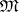satisfying a given system of polynomial identities. A variety of rings can be defined axiomatically as a hereditary class of algebras that is closed with respect to taking homomorphic images and complete direct sums (see Algebraic systems, variety of). Since the totality of polynomial identities that are satisfied in a given ring forms a fully-characteristic ideal (a-ideal) of a free ring, there exists a one-to-one correspondence between varieties of rings and the-ideals of a countably-generated free ring. If for two varieties of rings there is an inclusion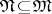, one says that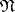is a subvariety of. The variety corresponding to the-ideal of identities of the ringis called the variety generated by the ring. Every variety of rings is generated by a "universal object" of it; this is a free ring of the given variety containing an infinite free system of generators: Every mapping from the set of free generators into an arbitrary ring of the variety can be extended to a homomorphism.
Let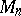be the variety generated by the algebra of square matrices of order. For each variety of associative rings of characteristic zero (that is, rings whose additive group is torsion-free) there exists a positive integersuch that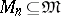, but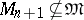. A variety of rings is called a Specht variety if every ring in it has a finite basis of identities. A variety generated by a finite associative ring or by a finite Lie ring is a Specht variety. The question whether every variety of associative algebras is a Specht variety forms the content of the Specht problem. If a varietyis generated by an associative algebra with a finite number of generators over a field of characteristic zero and if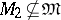, thenis a Specht variety.